﻿ Adding / Subtracting Multiples of 10 / 100 / 1000
 HOME ABOUT US SHOP PRIVACY CONTACT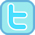# Adding / Subtracting Multiples of 10/100/1000+

 Mad Maths Minutes - Timed Drill & Fluency Builders Add / Subtract 10 (2-digit) Add multiple of 10 to 2-digit (within 100) Subtract multiple of 10 from 2-digit Add / Subtract multiple of 10 (within 100) Add / Subtract 100 (2/3-digit) Add multiple of 100 to 3-digit (within 1000) Subtract multiple of 100 from 3-digit Add / Subtract multiple of 100 Add / Subtract 1000 (within 10,000)
 Adding / Subtracting Powers of 10 Worksheets and Printables (.pdf)
 Our online activities...(to be updated in 2018)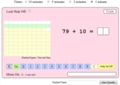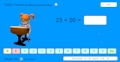Adding 10 to 2-digit Interactive Mad Maths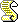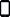Subtracting 10 from 2-digit Numbers Interactive Mad MathsAdding / Subtracting 10 (2-digit) InteractiveAdding multiples of 10 to 2-digit InteractiveSubtract multiples of 10 from 2-digit interactive mad mathsAdding / Subtracting Multiples of 10 (2-digit)Adding 100 to 3-digit Numbers InteractiveSubtract 100 from 3-digit InteractiveAdding/Subtracting 100 to/from 3-digit NumbersAdding multiples of 100 to 3-digit InteractiveSubtracting multiples of 100 from 3-digit InteractiveAdding / Subtracting Multiples of 100 InteractiveAdding multiples of 10 to 3-digit (multiples of 10) InteractiveAdding multiples of 10 to 3-digit InteractiveAdding 1000 InteractiveSubtract mulitple of 10 from 3-digit (multiple of 10) InteractiveSubtract multiples of 10 from 3-digit InteractiveSubtracting 1000 Interactive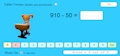Adding / Subtracting 2-digit and 3-digit multiples of 10Adding / Subtracting multiples of 10 and 3-digit numbers InteractiveAdding / Subtracting 1000 InteractiveAdding / Subtracting Powers of 10 (to 1000 000)Flash-Based Activities  & Links (Not compatible with some devices) Add 10 to 2-digit Alien Attack Add 10 to 2-digit Top of the Class Subtract 10 from 2-digit Alien Attack Add Mulitple of 10 to 2-digit Alien Attack